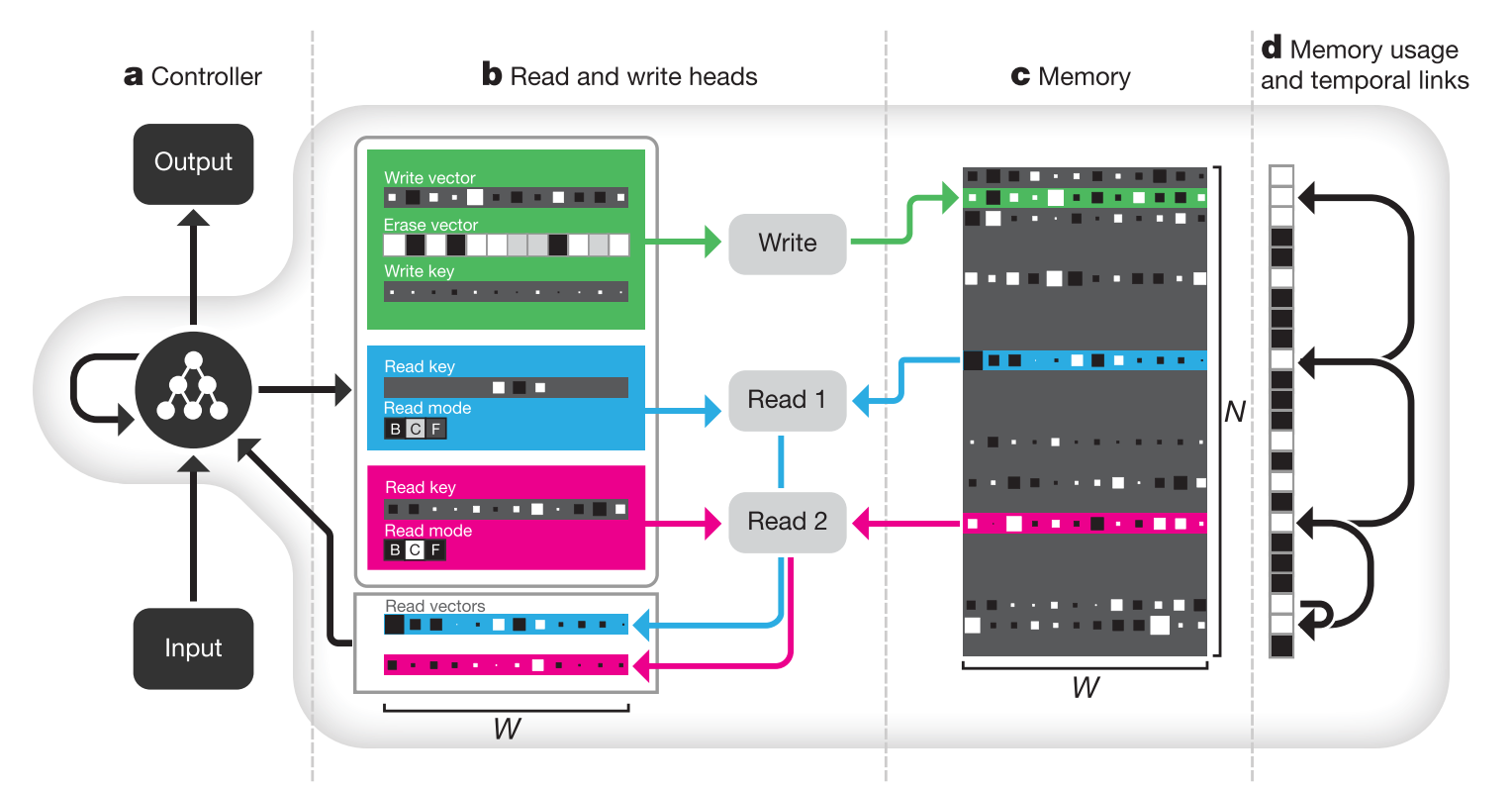# Hybrid computing using a neural network with dynamic external memory

## WHY?

Using external memory as modern computer enable neural net the use of extensible memory. This paper suggests Differentible Neural Computer(DNC) which is an advanced version of Neural Turing Machine.

## WHAT?

Reading and writing in DNC are implemented with differentiable attention mechanism.The controller of DNC is an variant of LSTM architecture that takes an input vector(x_t$x_t$) and a set of read vectors(r_{t-1}^1,...,r_{t-1}^R$r_{t-1}^1,...,r_{t-1}^R$) as input(concatenated). Concatenated input and hidden vectors from both previous timestep(h_{t-1}^l$h_{t-1}^l$) and from previous layer(h_t^{l-1}$h_t^{l-1}$) are concatenated again to be used as input for LSTM to produce next hidden vector(h_t^l$h_t^l$). Hidden vectors from all layers at a timestep are concatenated to emit an output vector(\upsilon_t$\upsilon_t$) and an interface vector(\xi_t$\xi_t$). The output vector(y_t$y_t$) is the sum of \upsilon_t$\upsilon_t$ and read vectors of the current timestep.

v_t = W_y[h_t^1;...;h_t^L]\\
\xi_t = W_{\xi}[h_t^1;...;h_t^L]\\
y_t = \upsilon_t + W_t[r_t^1;...;r_t^R]

THe interface vectors are consists of many vectors that interacts with memory: R read keys(\mathbf{k}_t^{r,i}\in R^W$\mathbf{k}_t^{r,i}\in R^W$), read strengths(\beta_t^{r,i}$\beta_t^{r,i}$), write key(\mathbf{k}_t^w\in R^W$\mathbf{k}_t^w\in R^W$), write strength(\beta_t^w$\beta_t^w$), erase vector(\mathbf{e}_t\in R^W$\mathbf{e}_t\in R^W$), write vector(\mathbf{v}_t\in R^W$\mathbf{v}_t\in R^W$), R free gates(f_t^i$f_t^i$), the allocation gate(g_t^a$g_t^a$), the write gate(g_t^w$g_t^w$) and R read modes(\mathbf{\pi}_t^i).

\mathbf{\xi}_t = [\mathbf{k}_t^{r,1};...;\mathbf{k}_t^{r,R};\beta_t^{r,1};...;\beta_t^{r,R};\mathbf{k}_t^w;\beta_t^w;\mathbf{e}_t;\mathbf{v}_t;f_t^1;...;f_t^R;g_t^a;g_t^w;\mathbf{\pi}_t^1;...;\mathbf{\pi}_t^R]

Read vectors are computed with read weights on memory. Memory matrix are updated with write weights, write vector and erase vector.

\mathbf{r}_t^i = M_t^T\mathbf{w}_t^{r,i}\\
M_t = M_{t-1}\odot(E-\mathbf{w}^w_t\mathbf{e}_t^T)+\mathbf{w}^w_t\mathbf{v}_t^T

Memory are addressed with content-based addressing and dynamic memory allocation. Contesnt-based addressing is basically the same as attention mechanism. Dynamic memory allocation is designed to clear memory as analogous to free list memory allocation scheme.

## So?Powered by aiden